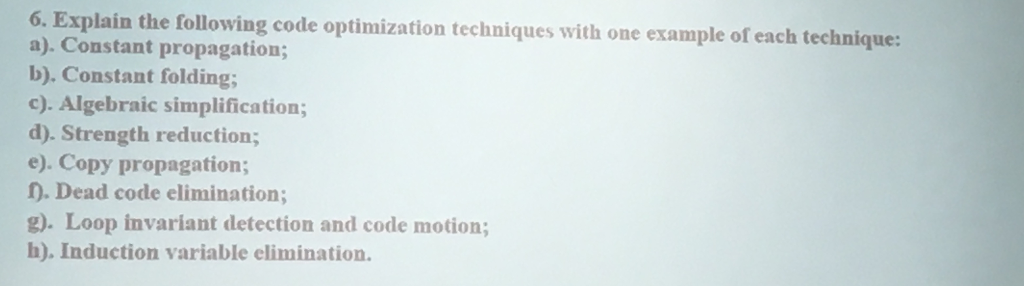# Question & Answer: Explain the following code optimization techniques with one example or each technique: a). Constant propagation:…..Explain the following code optimization techniques with one example or each technique: a). Constant propagation: b). Constant folding: c). Algebraic simplification: d). Strength reduction: e). Copy propagation: f). Dead code elimination: g). Loop invariant detection and code motion: h). Induction variable elimination.

1.constant propagation:

It is nothing but the variables are spread through (propagated)the flow graph and they substituted at the use of the variable.

Don't use plagiarized sources. Get Your Custom Essay on
Question & Answer: Explain the following code optimization techniques with one example or each technique: a). Constant propagation:…..
GET AN ESSAY WRITTEN FOR YOU FROM AS LOW AS \$13/PAGE

Let s take an example:

suppose x=3; and y=x+4;

Here to use of x the value of x can be propagated.

x=3 and y=7

After the constant propagation the above is the code.

Here you should aware that in more complex control flow some compilers will perform constant propagation and within basic blocks some compilers will perform.

2.Constant Folding

Expressions with constant operands can be evaluated at compile time, thus improving run-time performance and reducing code size by avoiding evaluation at compile-time.

At compile time expressions with constant operands can be checked and evaluated.This leads to improving of performance of run time and thus halps in reducing the size of the code by avoiding at compile time.

Example:

In the code fragment below, the expression (3 + 5) can be evaluated at compile time and replaced with the constant 8.

int f (void)
{
return 4 + 6;
}

In the above code the (4 +6) is the expression and can be evaluated at compile time and will be replaced with constant 10.

int f (void)
{
return 10;
}

So a bove is the code after constant folding.It is the easy optimation relatively.